Like   Tweet   in

# President's Note

 /* styles */ Dear NICA Community, While we are celebrating the start of the season for our fall leagues, as we are all well aware, this is not a normal race season. Fortunately, all of our leagues are able to have team and small group activities focused on practice, Teen Trail Corps work, Adventure rides, and more. Following local public health department guidelines, many fall leagues are doing individual time trial events where teams have allocated time frames and students are on course as individuals against the clock and two of our leagues are able to hold modified race events. We will miss our large community weekend events where we all gather to camp, enjoy one another's company, compete, and celebrate. Not to be, at least for now, but when it is safe for our athletes, coaches, volunteers, and staff to do so we will be back and stronger than ever. We know this because both student and coach registrations are up at the end of July compared to 2019, even with the COVID virus. We are needed more than ever for health and support and we will be here for you now and in the future, count on it! The reason NICA leagues are so successful, even in the midst of a pandemic, is our shared focus on the local interactions and experiences for our student-athletes. Our student-athletes are learning, like the rest of us, that life doesn’t always go as planned and how to readjust and make the most of what we can still do. I am so proud of all the great things that are happening for our student-athletes as our coaches continue to engage with them on the bike, safely interacting as a group or team, and having fun! We can continue our mission of getting more kids on bikes without races but we can not continue without the amazing effort, creativity, and dedication of our coaches and volunteers. Thank you to everyone for supporting NICA and the safety of our entire community during these unprecedented times. With Gratitude, Steve
 table div table+table+table+table div table{width:100%;padding:0}table div table+table+table+table div table img{width:96.23%;padding:0;float:none}table div table+table+table+table div table td{width:100%;padding:0 1.88% 18px}/* styles */# Wisconsin Coaches Adventure Training Weekend

The Wisconsin league held a Coaches Adventure training weekend at the Trek Headquarters in Waterloo, WI. Over 60 coaches participated in four sessions spread throughout the weekend. The coaches went through a progression of adventure activities that focused on map reading, orienteering, planning, and group problem solving. The intention of the weekend was to help coaches envision how to create adventure activities for their teams.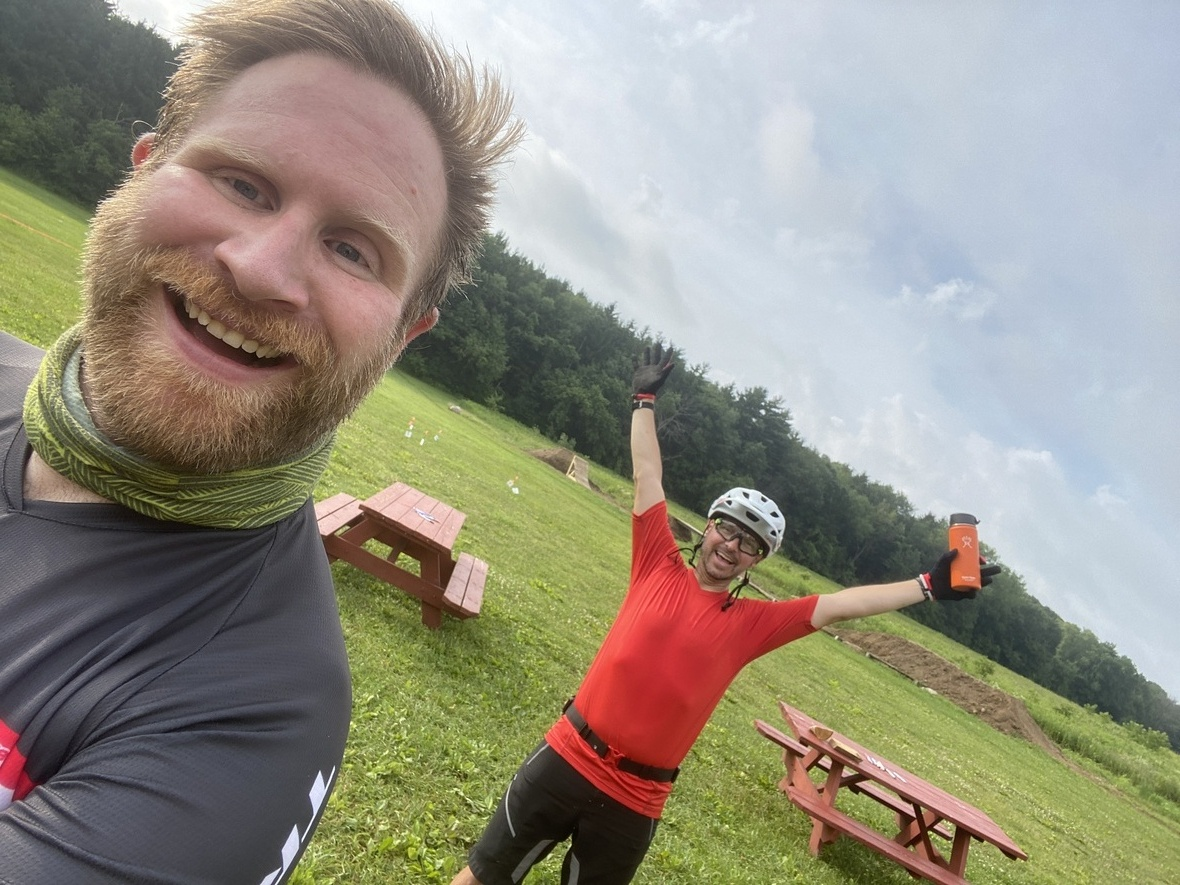Mike McGarry, NICA Coach Licensing Manager and Brian Heifner, WI Adventure Coordinator Photo Credit: Mike McGarry
 table div table+table+table+table+table+table+table+table div table{width:100%;padding:0}table div table+table+table+table+table+table+table+table div table img{width:96.23%;padding:0;float:none}table div table+table+table+table+table+table+table+table div table td{width:100%;padding:0 1.88% 18px}/* styles */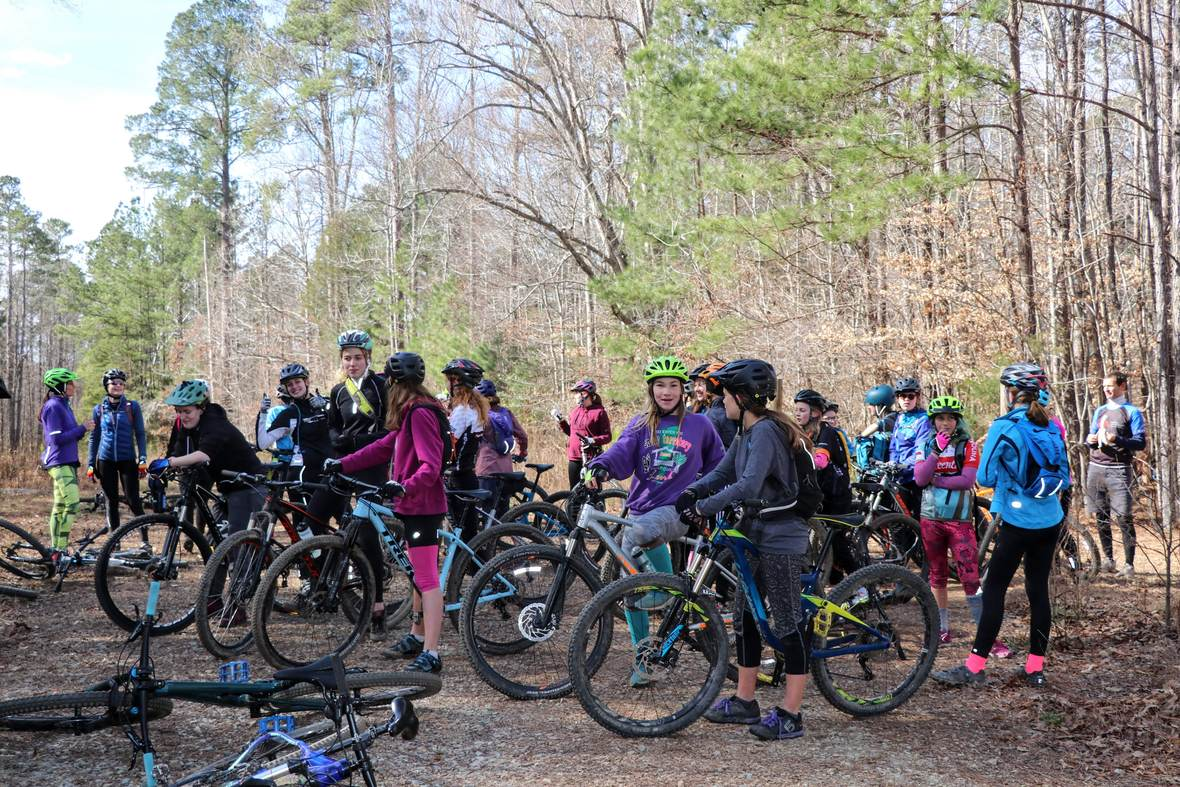November 2019, Brumley Forest, NC Photo Credit: Deborah Hage

# GRiT Regional Summits are a Success!

The Girls Riding Together Nationwide and Regional Summit sessions wrapped up at the end of July! GRiT Ambassadors, GRiT Coordinators, and GRiT Coaches came together via Zoom, representing their NICA state league, to discuss grassroot strategies to recruit female-identifying student-athletes. Everything from new GRiT tent activities to recruitment obstacles Ambassadors have faced. Talk to your league's GRiT Coordinator today to ask how you can get involved with your state's GRiT program. This initiative exists to move our female coach and student-athlete participation percentage from 20.4% nationwide to 33% by the year 2023.

The feedback we have been receiving from our GRiT Ambassadors about these first Summits has been so positive. One of the things we kept hearing was the desire for more mechanic-focused sessions next year. Not only are we getting #moregirlsonbikes, but we have more girls wanting to fix bikes too.

 table div table+table+table+table+table+table+table+table+table+table div table{width:100%;padding:0}table div table+table+table+table+table+table+table+table+table+table div table img{width:96.23%;padding:0;float:none}table div table+table+table+table+table+table+table+table+table+table div table td{width:100%;padding:0 1.88% 18px}/* styles */# Utah is off to the races!

On August 16th, the Utah League kicked off their season featuring the South Region at Soldier Hollow in Midway. Student-athletes raced in accordance with State and County Health Departments.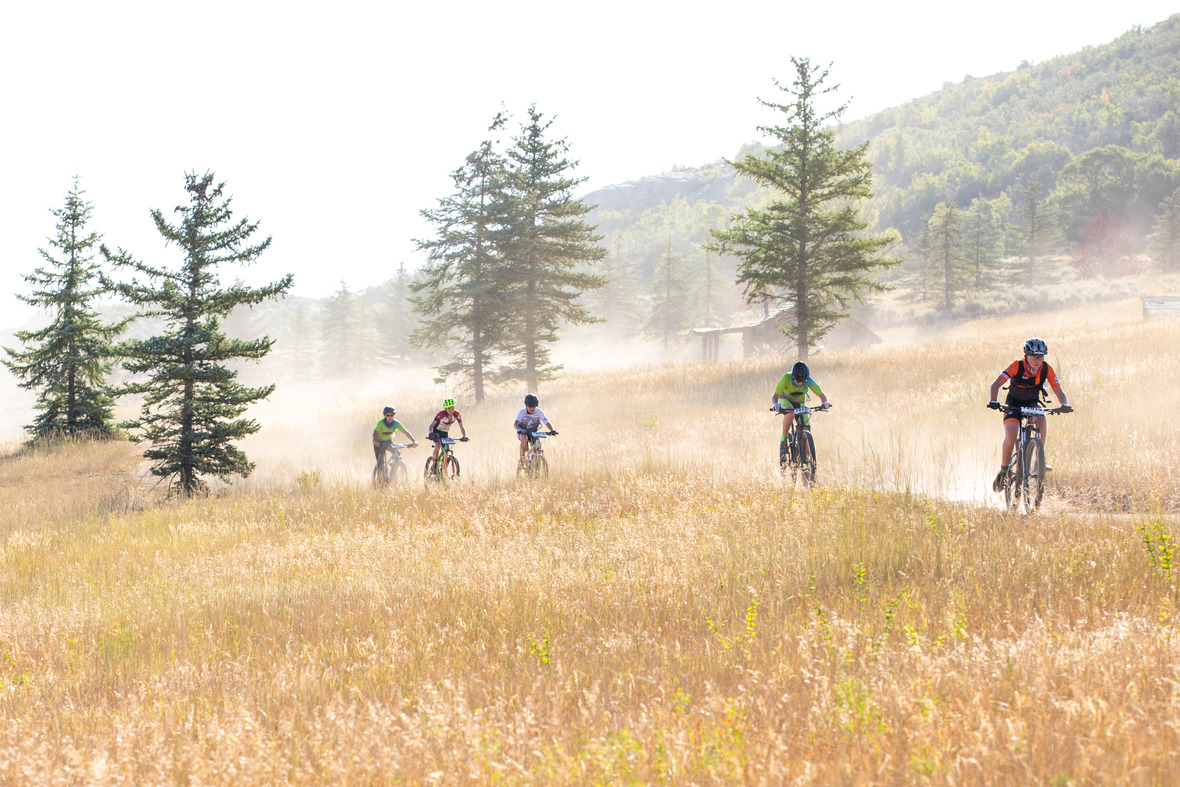Photo Credit: Angie Harker, Selective Vision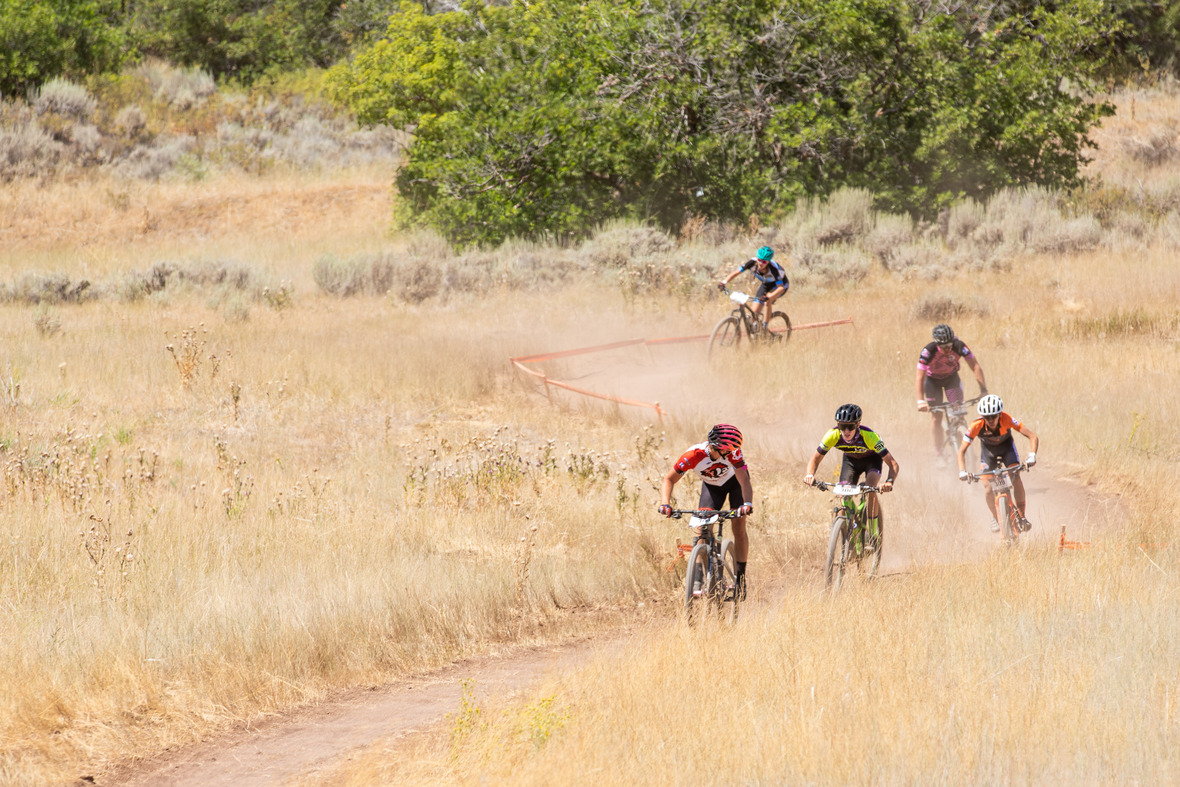Photo Credit: Angie Harker, Selective Vision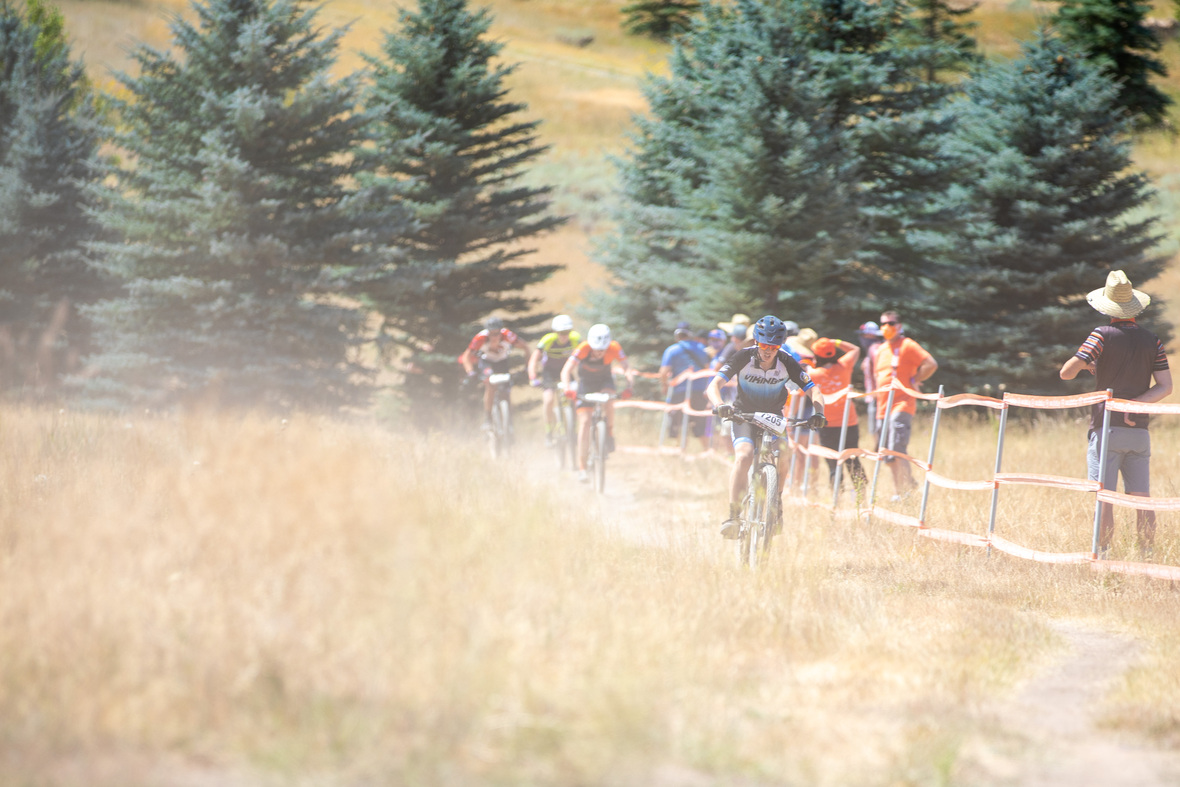Photo Credit: Angie Harker, Selective Vision
 table div table+table+table+table+table+table+table+table+table+table+table+table+table+table+table div table{width:100%;padding:0}table div table+table+table+table+table+table+table+table+table+table+table+table+table+table+table div table img{width:96.23%;padding:0;float:none}table div table+table+table+table+table+table+table+table+table+table+table+table+table+table+table div table td{width:100%;padding:0 1.88% 18px}/* styles */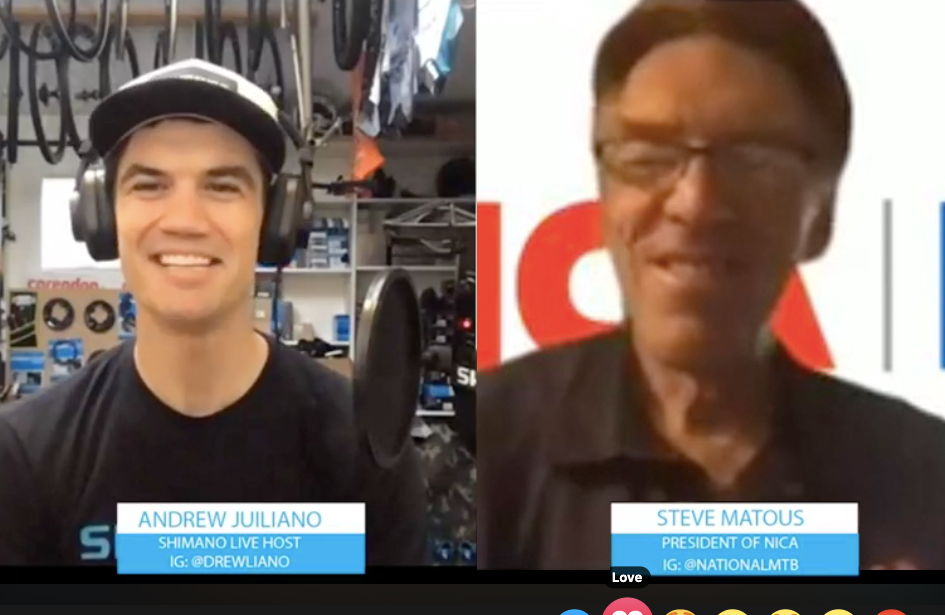Pro CX Racer Andrew Juiliano and NICA President Steve Matous

# Shimano LIVE Interview with Steve Matous

Shimano LIVE Host and Pro CX Racer Andrew Juiliano spoke with NICA President Steve Matous about getting more kids on bikes even during this exceptional time. In case you missed it, you can listen to it HERE.

 table div table+table+table+table+table+table+table+table+table+table+table+table+table+table+table+table+table div table{width:100%;padding:0}table div table+table+table+table+table+table+table+table+table+table+table+table+table+table+table+table+table div table img{width:96.23%;padding:0;float:none}table div table+table+table+table+table+table+table+table+table+table+table+table+table+table+table+table+table div table td{width:100%;padding:0 1.88% 18px}/* styles */# “Leave the Road, Take the Trails.” - Pythagore

Around the country we are excited by all of the new dirt in the works! As new leagues are established, local communities in those states are excited to welcome NICA and help ensure the league’s success. Lebanon and Terra Haute, IN, Shakopee, MN, and Florence, SC are just a few that have new trails in the works. Last month, new trails opened in West Virginia. Here’s the story:
In 2017, West Virginia League Director Cassie Smith and League Treasurer Steve Worden presented a modified Dirt Tour to all state park superintendents at the West Virginia Department of Natural Resources (WVDNR) Annual Conference. They had such a positive response to NICA’s mission to get more kids on bikes, that the WVDNR enthusiastically agreed to fund the design and construction of five amazing trails to be premiere mountain bike race venues throughout the state at select state parks. This summer, two more of these trails were opened up and ready to ride! These flow trails feature tight switchbacks, rock gardens, and swooping berms that will be sure to make riders smile for miles. This special relationship will benefit NICA and also the communities near the trails, providing access and open space to the public as well.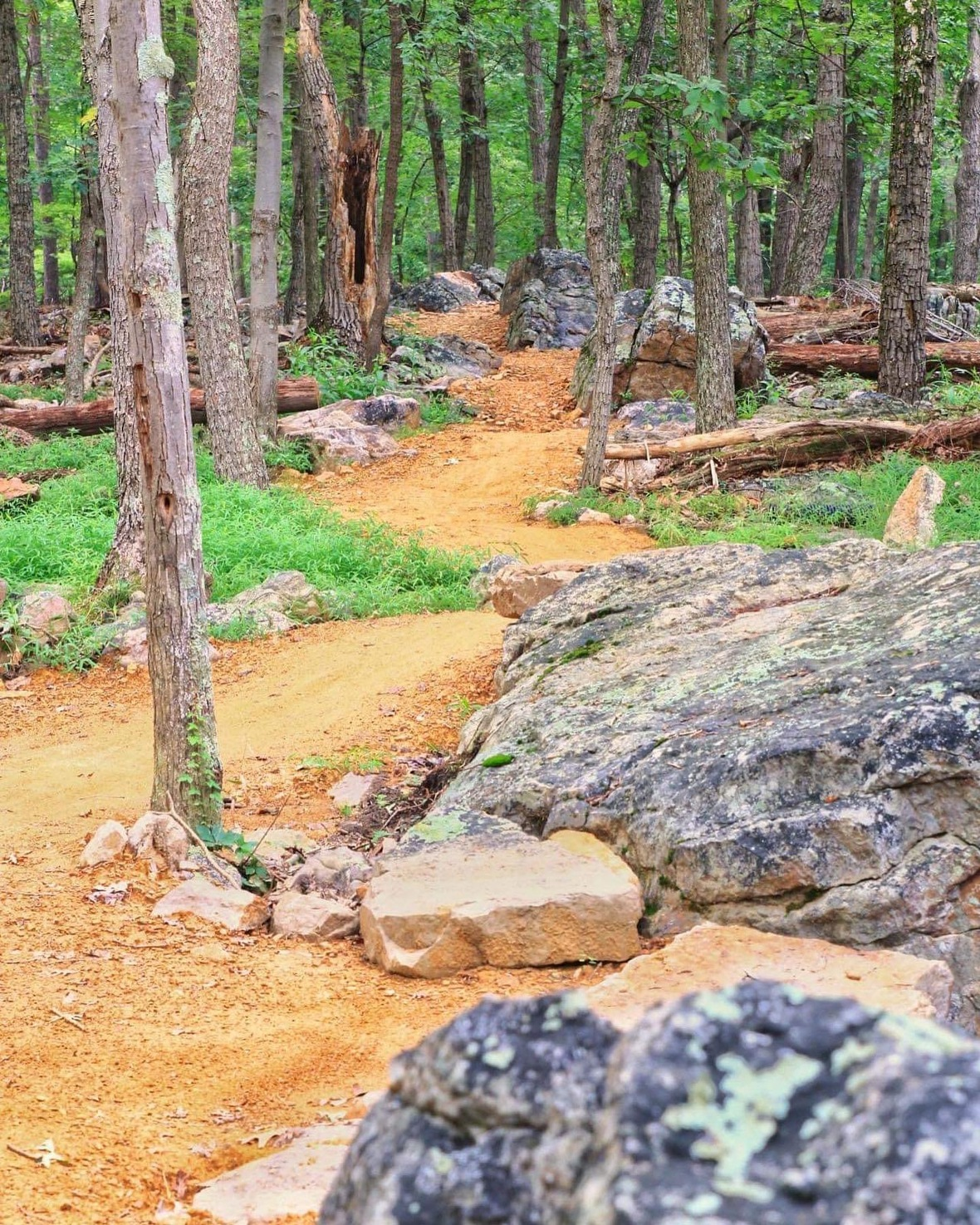Cacapon Resort State Park Photo Credit: Jack Hoblitzell Trail Builder: Appalachian Dirt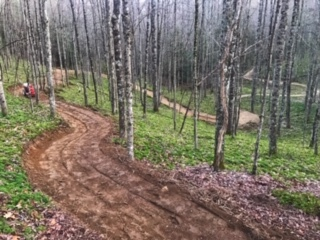Twin Falls Resort State Park Photo Credit: Tracy Toler Trail Builder: Adventure Trail Systems
 table div table+table+table+table+table+table+table+table+table+table+table+table+table+table+table+table+table+table+table+table div table{width:100%;padding:0}table div table+table+table+table+table+table+table+table+table+table+table+table+table+table+table+table+table+table+table+table div table img{width:96.23%;padding:0;float:none}table div table+table+table+table+table+table+table+table+table+table+table+table+table+table+table+table+table+table+table+table div table td{width:100%;padding:0 1.88% 18px}/* styles */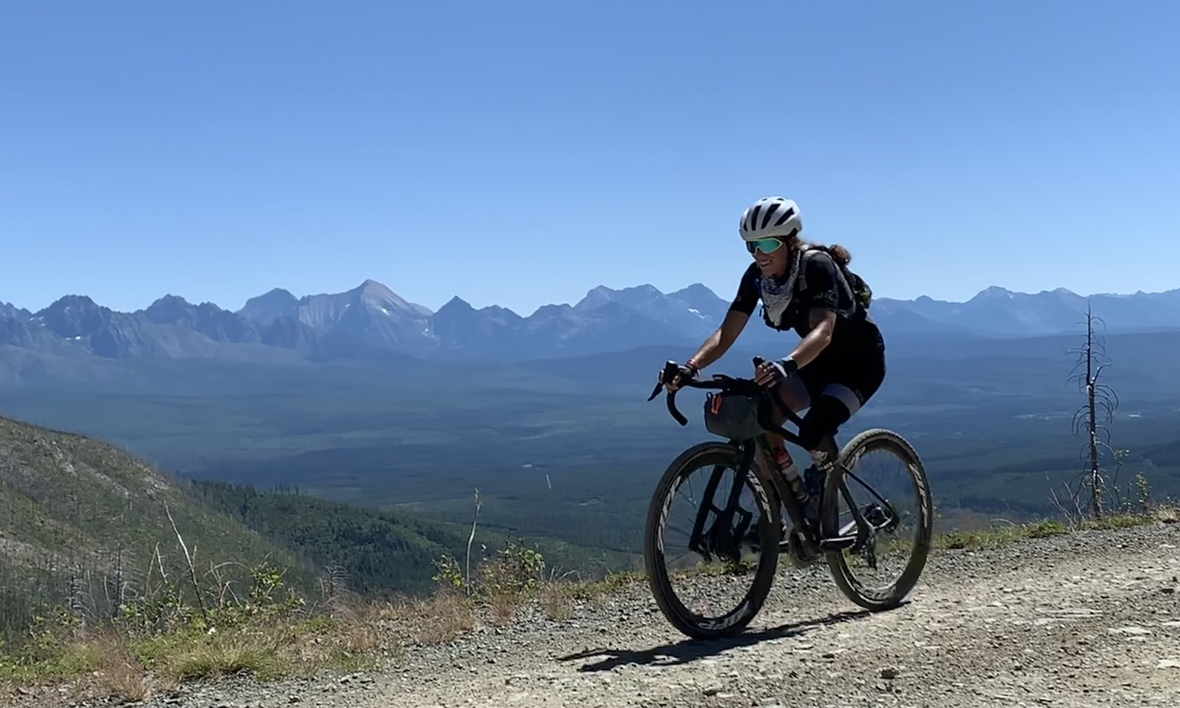World Champion and Paralympian Meg Fisher

# Leading By Example, A NICA Coach’s Empowering Story

Meg Fisher won Paralympic gold, silver and Bronze medals in the Rio 2016 and London 2012 games and ten world championships and is a NICA Coach for the Montana League.

Meg’s road to success has not been easy. At 19 years old she was involved in a tragic car accident that nearly killed her. She survived a coma and lost the lower portion of her left leg. Meg worked hard to heal and move beyond her new physical limitations.

Today, she is dedicated to helping erase limits, change lives, and demonstrate the capacity of the human body and spirit. “It’s a privilege to be a part of NICA this year. Missoula’s cycling community inspired and supported me for so many years and I feel very lucky for the opportunity to pay it forward to the next generation of riders,” says Meg Fisher.

Read her inspiring interview HERE. We are so happy to have her energy, experience and attitude on the NICA team.

 table div table+table+table+table+table+table+table+table+table+table+table+table+table+table+table+table+table+table+table+table+table+table div table{width:100%;padding:0}table div table+table+table+table+table+table+table+table+table+table+table+table+table+table+table+table+table+table+table+table+table+table div table img{width:96.23%;padding:0;float:none}table div table+table+table+table+table+table+table+table+table+table+table+table+table+table+table+table+table+table+table+table+table+table div table td{width:100%;padding:0 1.88% 18px}/* styles */# NICA 2019 Highlights

“NICA has truly become a nationwide movement, and we are incredibly grateful for the support that our sponsors, funders, volunteers, coaches, alumni and staff provide. Everyone who is part of the NICA community plays a key role in fulfilling our mission to build strong minds, bodies, characters and communities through cycling. Thank you for your dedication, investment and passion for helping us get #morekidsonbikes, says NICA Board Chair Susan Helm-Murtagh.” You can view our Annual Report, NICA 2019 Highlights HERE.

 table div table+table+table+table+table+table+table+table+table+table+table+table+table+table+table+table+table+table+table+table+table+table+table+table div table{width:100%;padding:0}table div table+table+table+table+table+table+table+table+table+table+table+table+table+table+table+table+table+table+table+table+table+table+table+table div table img{width:96.23%;padding:0;float:none}table div table+table+table+table+table+table+table+table+table+table+table+table+table+table+table+table+table+table+table+table+table+table+table+table div table td{width:100%;padding:0 1.88% 18px}/* styles */table div table+table+table+table+table+table+table+table+table+table+table+table+table+table+table+table+table+table+table+table+table+table+table+table+table div table td,table.module-24{width:100%;padding:0}table div table+table+table+table+table+table+table+table+table+table+table+table+table+table+table+table+table+table+table+table+table+table+table+table+table div table{width:100%;float:none;margin-left:auto;margin-right:auto;padding:0}table div table+table+table+table+table+table+table+table+table+table+table+table+table+table+table+table+table+table+table+table+table+table+table+table+table div table a{border:0 none;text-decoration:none}table div table+table+table+table+table+table+table+table+table+table+table+table+table+table+table+table+table+table+table+table+table+table+table+table+table div table img{width:100%!important;border:0 none;text-decoration:none}/* styles */
 table div table+table+table+table+table+table+table+table+table+table+table+table+table+table+table+table+table+table+table+table+table+table+table+table+table+table div table{width:100%;padding:0}table div table+table+table+table+table+table+table+table+table+table+table+table+table+table+table+table+table+table+table+table+table+table+table+table+table+table div table img{width:96.23%;padding:0;float:none}table div table+table+table+table+table+table+table+table+table+table+table+table+table+table+table+table+table+table+table+table+table+table+table+table+table+table div table td{width:100%;padding:0 1.88% 18px}/* styles */# NICA is Great NonProfits 2020 Top Rated

Thanks to our strong community, we are a 2020 Top Rated organization with Great Nonprofits. This acknowledgement is incredibly meaningful because the reviews are crowdsourced. We are grateful that so many of you gave us high marks!

 table div table+table+table+table+table+table+table+table+table+table+table+table+table+table+table+table+table+table+table+table+table+table+table+table+table+table+table+table div table{width:100%;padding:0}table div table+table+table+table+table+table+table+table+table+table+table+table+table+table+table+table+table+table+table+table+table+table+table+table+table+table+table+table div table img{width:96.23%;padding:0;float:none}table div table+table+table+table+table+table+table+table+table+table+table+table+table+table+table+table+table+table+table+table+table+table+table+table+table+table+table+table div table td{width:100%;padding:0 1.88% 18px}/* styles */# Host a Fundraiser for your team with Filmed by Bike

Host a Fundraiser for your team with Filmed by Bike
Filmed by Bike is a film festival that features the world’s best bike movies. To support organizations like NICA, they offer a touring program with two family-friendly collections of films that you can show as a fundraiser for your team.

The films in the collections feature positive stories featuring all body types, plenty of women on bikes and Black, Indigenous and all People of Color. Learn more about how you can host your own fundraiser HERE.

 table div table+table+table+table+table+table+table+table+table+table+table+table+table+table+table+table+table+table+table+table+table+table+table+table+table+table+table+table+table+table div table{width:100%;padding:0}table div table+table+table+table+table+table+table+table+table+table+table+table+table+table+table+table+table+table+table+table+table+table+table+table+table+table+table+table+table+table div table img{width:96.23%;padding:0;float:none}table div table+table+table+table+table+table+table+table+table+table+table+table+table+table+table+table+table+table+table+table+table+table+table+table+table+table+table+table+table+table div table td{width:100%;padding:0 1.88% 18px}/* styles */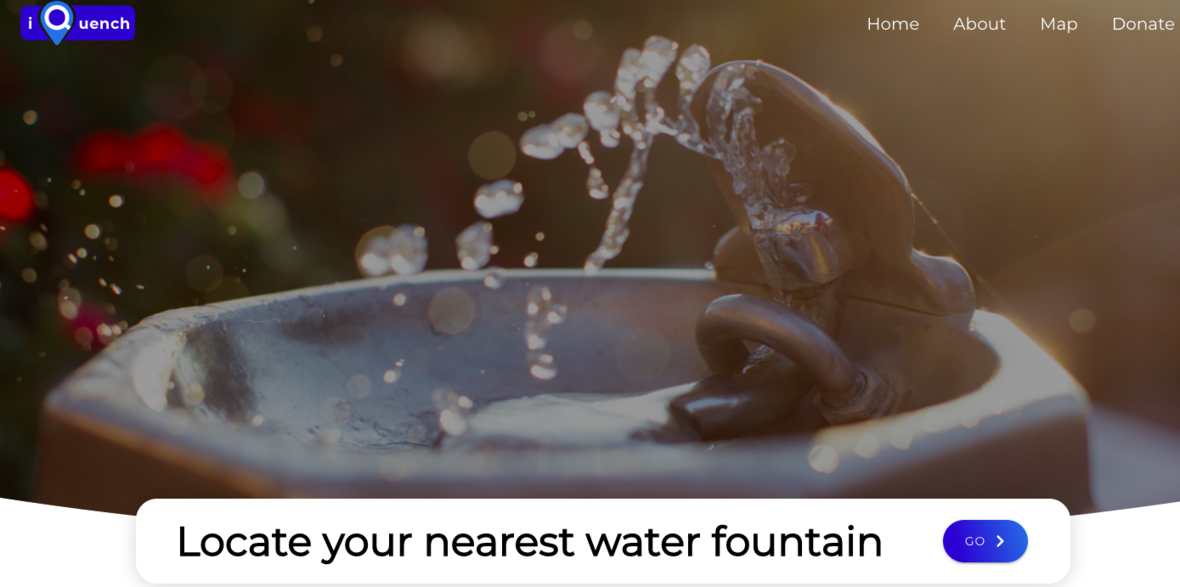iQuench was started by NICA Student-Athlete Milan Ferus-Comelo

# A new way to find water on your ride - iQuench

The creativity of our student-athletes is phenomenal. They keep coming up with innovative solutions to the problems they see. iQuench, started by Milan Ferus-Comelo of our NorCal League, identifies drinking fountain locations that are crowd-sourced from all over the country. The app shows nearby water fountains and can give directions on how to get there. For example, if you're out on a ride and run out of water, all you need to do is pull up the app and find one that's close by. To handle the problem of so many fountains being closed due to the coronavirus, iQuench clearly displays which fountains are not working with greyed-out icons.

 table div table+table+table+table+table+table+table+table+table+table+table+table+table+table+table+table+table+table+table+table+table+table+table+table+table+table+table+table+table+table+table+table div table{width:100%;padding:0}table div table+table+table+table+table+table+table+table+table+table+table+table+table+table+table+table+table+table+table+table+table+table+table+table+table+table+table+table+table+table+table+table div table img{width:96.23%;padding:0;float:none}table div table+table+table+table+table+table+table+table+table+table+table+table+table+table+table+table+table+table+table+table+table+table+table+table+table+table+table+table+table+table+table+table div table td{width:100%;padding:0 1.88% 18px}/* styles */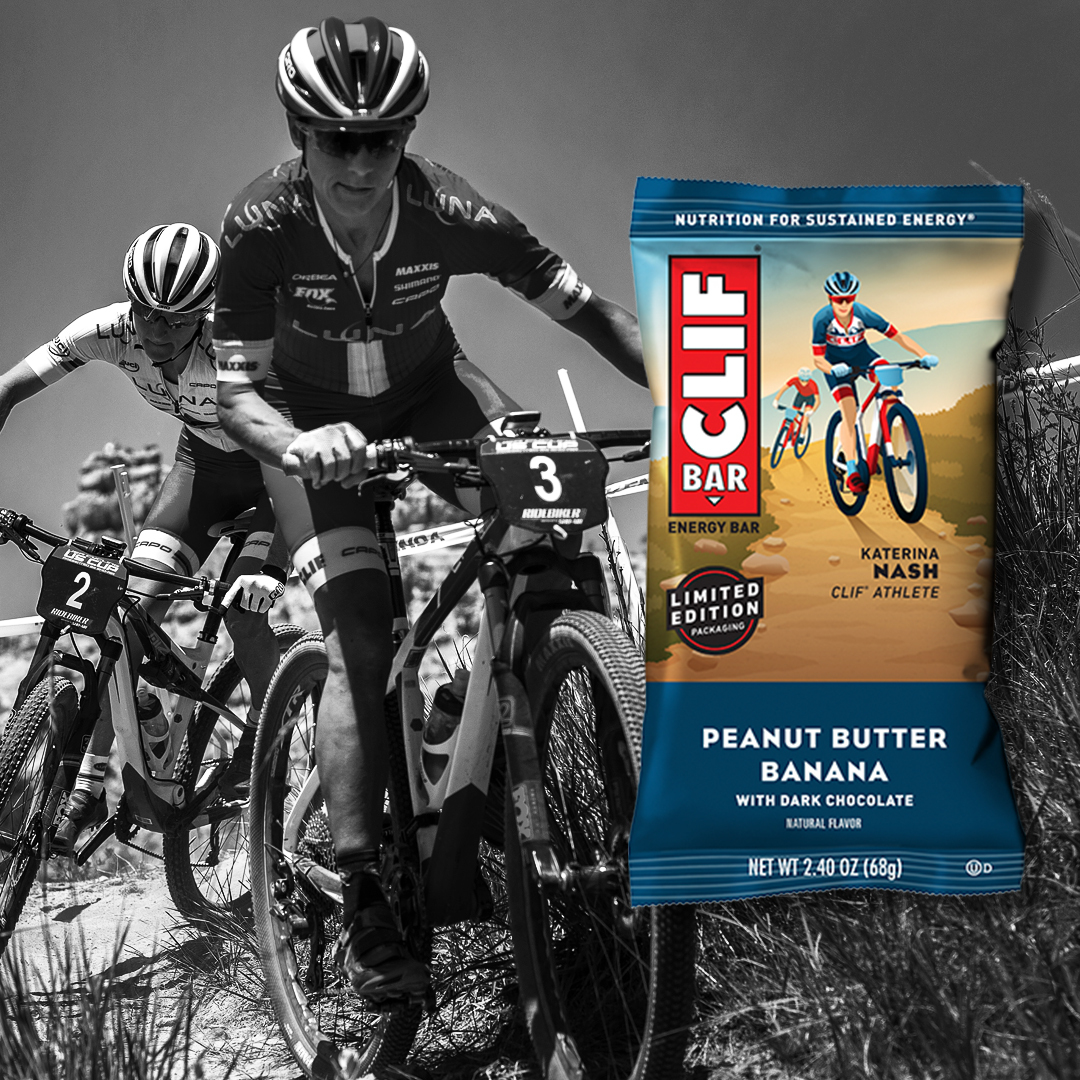5-time Olympian & CLIF Athlete Katerina Nash

# CLIF Bars Celebrates Women Athletes

Sustain your energy with limited-edition CLIF Bars, celebrating the achievements of six trailblazing and world champion women athletes, including cyclist Katerina Nash; a 5-time Olympian & CLIF Athlete since 2002. The idea for CLIF BAR® was born on a 175-mile bike ride. Katerina Nash doesn’t have to ride quite that far, but the sustained energy found in Peanut Butter Banana with Dark Chocolate CLIF BAR® is perfect for helping her power through long days on the course. Find Katerina’s limited-edition packaging, as well as 5 other amazing female athletes at www.clifbar.com.

 table div table+table+table+table+table+table+table+table+table+table+table+table+table+table+table+table+table+table+table+table+table+table+table+table+table+table+table+table+table+table+table+table+table+table div table{width:100%;padding:0}table div table+table+table+table+table+table+table+table+table+table+table+table+table+table+table+table+table+table+table+table+table+table+table+table+table+table+table+table+table+table+table+table+table+table div table img{width:96.23%;padding:0;float:none}table div table+table+table+table+table+table+table+table+table+table+table+table+table+table+table+table+table+table+table+table+table+table+table+table+table+table+table+table+table+table+table+table+table+table div table td{width:100%;padding:0 1.88% 18px}/* styles */# New Spherical Technology from Bell+Giro

Helmets with Spherical Technology provide comprehensive protection and performance. Developed at the Bell+Giro Dome in partnership with MIPS®, Spherical Technology’s unique ball-and-socket design is powered by the MIPS Brain Protection System to redirect impact forces, bringing market leading design, performance and brain protection together without compromising comfort, ventilation, weight or style. This acclaimed head protection technology is available in Giro’s premier trail riding helmet, the Manifest Spherical, as well as the Aether Spherical and Tyrant Spherical helmets.

 table div table+table+table+table+table+table+table+table+table+table+table+table+table+table+table+table+table+table+table+table+table+table+table+table+table+table+table+table+table+table+table+table+table+table+table+table div table{width:100%;padding:0}table div table+table+table+table+table+table+table+table+table+table+table+table+table+table+table+table+table+table+table+table+table+table+table+table+table+table+table+table+table+table+table+table+table+table+table+table div table img{width:96.23%;padding:0;float:none}table div table+table+table+table+table+table+table+table+table+table+table+table+table+table+table+table+table+table+table+table+table+table+table+table+table+table+table+table+table+table+table+table+table+table+table+table div table td{width:100%;padding:0 1.88% 18px}/* styles */# New GU Liquid Energy Gels

GU Liquid Energy Gels are now available! Liquid Energy Gels are the GU YOU CAN GULP. This is a new form factor that delivers the same key ingredients as our original Energy Gels, but in a liquid. Caffeine free options include Lemonade & Strawberry Banana.

 table div table+table+table+table+table+table+table+table+table+table+table+table+table+table+table+table+table+table+table+table+table+table+table+table+table+table+table+table+table+table+table+table+table+table+table+table+table+table div table{width:100%;padding:0}table div table+table+table+table+table+table+table+table+table+table+table+table+table+table+table+table+table+table+table+table+table+table+table+table+table+table+table+table+table+table+table+table+table+table+table+table+table+table div table img{width:96.23%;padding:0;float:none}table div table+table+table+table+table+table+table+table+table+table+table+table+table+table+table+table+table+table+table+table+table+table+table+table+table+table+table+table+table+table+table+table+table+table+table+table+table+table div table td{width:100%;padding:0 1.88% 18px}/* styles */# Ready to Ride?

Checkout the latest blog post on bike safety from our friends at Feedback Sports HERE! Happy trails everyone!

 table div table+table+table+table+table+table+table+table+table+table+table+table+table+table+table+table+table+table+table+table+table+table+table+table+table+table+table+table+table+table+table+table+table+table+table+table+table+table+table+table div table{width:100%;padding:0}table div table+table+table+table+table+table+table+table+table+table+table+table+table+table+table+table+table+table+table+table+table+table+table+table+table+table+table+table+table+table+table+table+table+table+table+table+table+table+table+table div table img{width:96.23%;padding:0;float:none}table div table+table+table+table+table+table+table+table+table+table+table+table+table+table+table+table+table+table+table+table+table+table+table+table+table+table+table+table+table+table+table+table+table+table+table+table+table+table+table+table div table td{width:100%;padding:0 1.88% 18px}/* styles */# NICA exists by virtue of our generous sponsors, boosters, foundation partners and individual donors.

We are extremely grateful for your continued support of our mission to build strong minds, bodies, character and communities through cycling. Consider supporting NICA or your favorite league today and help us impact the future of cycling!

 table div table+table+table+table+table+table+table+table+table+table+table+table+table+table+table+table+table+table+table+table+table+table+table+table+table+table+table+table+table+table+table+table+table+table+table+table+table+table+table+table+table+table+table div table{width:100%;padding:0}table div table+table+table+table+table+table+table+table+table+table+table+table+table+table+table+table+table+table+table+table+table+table+table+table+table+table+table+table+table+table+table+table+table+table+table+table+table+table+table+table+table+table+table div table img{width:96.23%;padding:0;float:none}table div table+table+table+table+table+table+table+table+table+table+table+table+table+table+table+table+table+table+table+table+table+table+table+table+table+table+table+table+table+table+table+table+table+table+table+table+table+table+table+table+table+table+table div table td{width:100%;padding:0 1.88% 18px}/* styles */table.module-43{width:75.47%;padding:0}table div table+table+table+table+table+table+table+table+table+table+table+table+table+table+table+table+table+table+table+table+table+table+table+table+table+table+table+table+table+table+table+table+table+table+table+table+table+table+table+table+table+table+table+table div table{width:75.47%;float:none;margin-left:auto;margin-right:auto;padding:0}table div table+table+table+table+table+table+table+table+table+table+table+table+table+table+table+table+table+table+table+table+table+table+table+table+table+table+table+table+table+table+table+table+table+table+table+table+table+table+table+table+table+table+table+table div table a{border:0 none;text-decoration:none}table div table+table+table+table+table+table+table+table+table+table+table+table+table+table+table+table+table+table+table+table+table+table+table+table+table+table+table+table+table+table+table+table+table+table+table+table+table+table+table+table+table+table+table+table div table img{width:100%!important;border:0 none;text-decoration:none}table div table+table+table+table+table+table+table+table+table+table+table+table+table+table+table+table+table+table+table+table+table+table+table+table+table+table+table+table+table+table+table+table+table+table+table+table+table+table+table+table+table+table+table+table div table td{width:100%;padding:0}/* styles */